# PSEB 10th Class Science Important Questions Chapter 12 Electricity

Punjab State Board PSEB 10th Class Science Important Questions Chapter 12 Electricity Important Questions and Answers.

## PSEB 10th Class Science Important Questions Chapter 12 Electricity

Question 1.
(a) What is meant by Joule’s heating effect due to flow of current through a conductor?
The conductors offer some resistance to the flow of current. If I is the current in ampere flowing for t second, then quantity of charge Q = I × t.

The work done in carrying a charge Q ( It). coulomb against a potential difference of
1 volt. W = V × Q
or W = V × It
= VIt joule

The whole of this energy is converted into heat.
∴ Heat produced, H = W = VIt joule ……….. (i)

Now V = IR
H = (IR)It joule
or H = I2Rt joule ………. (ii)
Again
I = $$\frac{V}{R}$$
∴ H = $$\frac{\mathrm{V}^{2}}{\mathrm{R}^{2}}$$ Rt joule
or H = $$\frac{\mathrm{V}^{2}}{\mathrm{R}}$$ t joule ………….. (iii)

Thus, when current is passed through a conductor, the heat produced is given by;
H = VIt = I2Rt = $$\frac{\mathrm{V}^{2}}{\mathrm{R}}$$ t
and is called Joules heating effect.

(b) Define electric power and unit of electric power.
Electric Power: The rate of doing electric work is called electric power. Suppose ‘V’ is the potential difference between the ends of a conductor and T is the current flowing through it,

Work done by flow of current T for ‘t’ second, W = VIt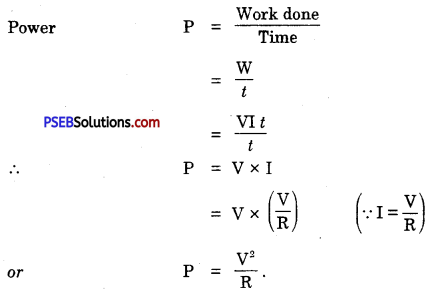Unit of Electric Power. We know P = V x I. If potential difference V is measured in volt and current T in ampere than power will be in watt.
1 watt = 1 volt x 1 ampere

The bigger unit of power is Kilowatt (kw)
1 kilowatt = 1000 watt.

(c) What do you mean by electric energy? Give the definition of its unit.
Electric Energy: Total work done by electric current in a fixed time is called electric energy.

Suppose I ampere of current flows through a conductor for t seconds when the potential difference across the ends of a conductor is V, then total work done or electric energy consumed,
E = W = V × I × t

The unit of electric energy is joule or watt-second but this is a small unit.
The bigger unit of electric energy is watt hour.

Watt hour. The electric energy consumed in a circuit is said to be watt hour if 1 ampere of current flows for 1 hour and potential differenc across the ends is 1 volt.
1 watt-hour = 1 watt × 1 hr.
= 1 volt × l ampere × 1 hour 1 kilowatt hour (kwh) = 1000 watt hours
The bigger (commercial) unit of electric energy is kilowatt hour (kwh).

Question 2.
What is meant by resistance of a conductor? On what factors does the resistance of conductor depends?
Resistance of Conductor. It is defined as the ratio of potential difference across its ends to the current flowing through the conductor is called resistance of the conductor. It is denoted by ‘R’.

If V is the potential difference between the ends of the conductor and T is the current flowing through the conductor, then
Resistance (R) = $$\frac{\text { Potential Difference }(\mathrm{V})}{\text { Current }(\mathrm{I})}$$

Unit of Resistance. S.I. unit of resistance is ohm.

Factors on which resistance of conductor depends.

• Electric source (i.e. battery or cell)
• Conductor
• Switch (or key)
• Any other instrument connected in the circuit.

Question 3.
Find experimentally the various factors on which resistance of conductor depends.
On what factors the resistance of a conductor depends is shown by the following experiment :

Experiment: Arrange an electric circuit consisting of a battery, ammeter, connecting wire and conductor with the help of a switch (key). Press the switch and allow the current to flow through the circuit. Note the value of current from the ammeter. Now in place of this wire, connect another wire of place of this wire, connect another wire of same length and thickness and note the reading of ammeter. You will find that the value of current changes. This experiment shows that resistance of conductor depends upon the nature of material of wire i.e. at the same temperature the resistance of different conductors of same length and thickness is different.

Now take a wire of the same material as that of the first conductor and same diameter but of double length. Connect this wire in the same circuit and allow the current to flow through it. You will see this value is half of the first value. This shows that resistance is proportional to the length. If R is the resistance of the conductor and l the length then,
R ∝ l …(i)
Now take two wires of the same material but of different areas of cross section. At first connect a wire of small cross-section in the circuit and then replace it with a wire of large area of cross-section. Note the value of current I in two cases. You will see that more current flows in the second wire than in the first wire. It is clear that resistance of second wire is less than the first wire.
i.e. R ∝$$\frac{1}{A}$$ ……(ii)

Combining (i) and (ii), we get
R ∝$$\frac{l}{A}$$ …..(iii)
or R = ρ. $$\frac{l}{A}$$ …(iv)
where ρ is a constant and is called resistivity of the conductor. Its value depends upon the nature of material of conductor (wire).

Question 4.
What is Ohm’s law? How can it be verified?
Or
Write ohm’s law. Draw a circuit diagram to prove it experimentally in the laboratory,
Ohm’s law. It states that the current passing through a conductor is directly proportional to the potential difference across its ends provided the temperature and other physical conditions remains unchanged.
I ∝ Y
i.e. V ∝ I
or V = RI
Where R is a constant of proportionalty called resistance.

Resistance. It is the property of a conductor to oppose the flow of current. Resistance depends upon the nature of the conductor, its temperature and its dimensions (length, area).
R = $$\frac{V}{I}$$
or I = $$\frac{V}{R}$$
Experimental Verification of Ohm’s Law: Connect an ammeter, battery, key and rheostat as shown in Fig. 12.7(a). Put the plug in the key K. Read the values of potential difference across resistor R with the help of voltmeter V and the current flowing through the resistor with the help of ammeter.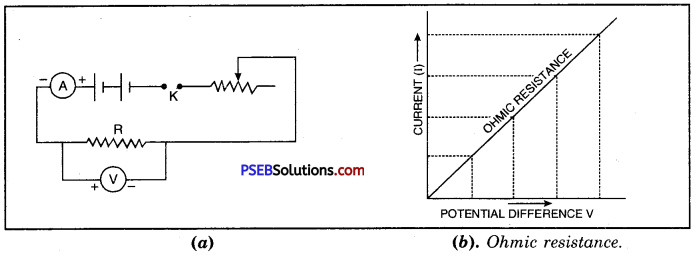Note the readings. Vary the current in the circuit by sliding contact of rheostat and go on noting reading in voltmeter and ammeter. Take the ratio potential difference (V) and corresponding value of current (I) each time. The ratio would be same, which proves Ohm’s law. Now plot a graph between V and I on graph paper. It will be straight line graph as shown in Figure (b) which also verifies that V ∝ I.Question 5.
What is the need of combining different resistors? What is the resultant resistance when a number of resistances are connected in series?
Or
State Ohm’s law. Find the equivalent of resistances of the individual resistances connected in series.
Ohm’s law: It states that the current passing through a conductor is directly proportional to the potential difference across its ends provided the temperature and other physical conditions remains unchanged.
I ∝ Y
i.e. V ∝ I
or V = RI
Where R is a constant of proportionalty called resistance.

Combination of Resistors: Resistors of all values of resistances are not available. Hence resistors are connected in a number of ways to increase or decrease the combined resistance. There are two distinct ways in which resistors can be connected. They are (i) resistors in series and (ii) resistors in parallel.

Resistors Connected In Series: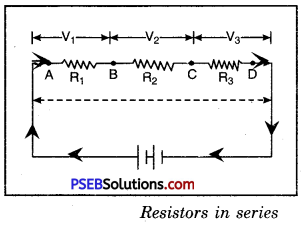Resistors are said to be connected in series, if they are joined end to end so that the same current flows through each one of them in succession. Let the resistors R1( R2 and R3, be joined in series and let the current passing through them be I [Figure].

Let V1, V2, and V3 be the potential difference between the ends of the first, second and third resistor respectively.
By Ohm’s law,
V1 = IR1; V2 = IR2 and V3 = IR3

If V is the total potential difference between the ends A and D and R is the effective resistance of the combination of all the resistors, then
V = IRS
But V = V1 + V2 + V3.
or IRs = IR1 + IR2 + IR3
IRs = I(R1 + R2 + R3)
or Rs = R1 + R2 + R3

The above result holds good for any number of resistors joined in series.
Thus when resistors are joined in series, the total resistance is equal to the sum of individual resistances.

Question 6.
With the help of a diagram derive the formula for the equivalent resistance of three resistances connected in parallels.
Or
With the help of a labelled circuit diagram derive a formula to find combined resistance (R) when two or more resistances (R1, R2, R3) are connected in parallel taking symbols potential difference (V) and current (I).
A circuit in which two or more resistors are connected across two common points so as to provide separate paths is called parallel circuit.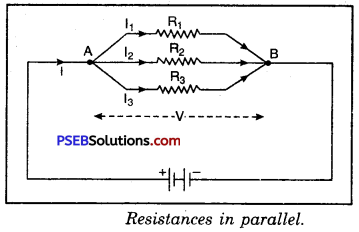In this case, the same potential difference will be maintained between the two ends of every resistor and the current will divide itself in various branches.

Let the resistors R1; R2 and R3 be joined in parallel between the points A and B.
Let the current I reaching A divide itself into three parts I1; I2 and I3 along R1, R2 and R3 respectively. Let V be the potential difference between the points A and B.

The currents flowing in the individual resistors are then given by :
I1 = $$\frac{V}{R_{1}}$$,
I2 = $$\frac{V}{R_{2}}$$ and
I3 = $$\frac{V}{R_{3}}$$
Let Rp be the resistance of the combination,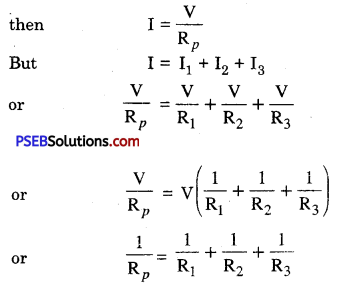The result holds good for any number of resistances.

Question 7.
What is meant by electric power? Give its units. Also give its SI unit.
Or
What is power? Give commercial unit of power.
Or
What is electric energy? What is its SI unit?
Power is the rate of doing work. The electric power of an appliance is its rate of consumption of electric energy.
The power of an electric appliance is 1 W (watt) if it consumes 1 J (joule) of energy in 1 second.

If I ampere of the current flows for t second through a coil whose ends are maintained at a potential difference of V volt, then the energy consumed or the work done.
W = VIt joule

Power = $$\frac{\mathrm{W}}{t}$$
= $$\frac{\mathrm{VI} t \text { joule }}{t \text { second }}$$
or P = V × I joule/second
or = VI watt
∴ P (watt) = V (volt) × J (ampere)
or watt = volt × ampere

Bigger unit of power is usually kW (kilowatt)
1 kW = 1,000 W
P(in kW) = $$\frac{\text { watt }}{1,000}=\frac{\mathrm{V} \text { (volt) } \times \mathrm{I} \text { (ampere) }}{1,000}$$

Electric energy is the total amount of work done by the current in a given time or electric energy is the total amount of energy consumed in an electric circuit in a given time.
W (joule) = P(watt) × t (second)
or W = P$$\left(\frac{\mathrm{J}}{\mathrm{s}}\right)$$ × t (s)
= P × t joule
But P = VI
∴ W = VIt joule

Practical Unit of Electric Energy: Practical unit of electric energy called kWh (kilowatt-hour) is usually used. This unit is also called B.O.T. (Board of Trade Unit).

The unit kWh is equal to the work done or energy consumed when a power of IkW is consumed for 1 hour.
Energy in kWh = $$\frac{\text { watt } \times \text { hour }}{1,000}$$
or
Energy in kWh = $$\frac{\text { volt } \times \text { ampere } \times \text { hour }}{1,000}$$

Question 1.
What is the contribution of electricity in our daily life?
Contribution of Electricity in our Life. Electricity finds great contribution in our life. It gives many facilities like its use in electric bulb and tubelight removes darkness at night, in summers with its use desert coolers and air conditioners keep our houses cool while in winters with its use in heaters we keep our houses warm. Besides this electricity is used in televisions, radios and cinemas for our entertainment. Electricity is also used to work the various machines the fields of agriculture, transport and industry.

Question 2.
What do you understand by static electricity?
Static Electricity: When two bodies are rubbed against each other then they acquire a property to attract lighter bodies towards them that is they get static charge. Electricity produced by rubbing the two bodies with each other is called frictional electricity or static electricity. And the study of static charges is called electrostatics.

Example: When a plastic pen is rubbed with dry hair then the pen attracts small bits of paper towards itself. This is due to the electricity produced by rubbing.Question 3.
What are positive and negative charges? How are these produced?
Positive charge: The charge produced in glass rod when it is rubbed with silk cloth is called positive charge.
Negative charge: The charge produced on ebonite rod when it is rubbed with cat skin is called negative charge.

Question 4.
What is an electric circuit?
Electric circuit. It is a closed path through which the electrons flow readily. When a conductor is connected to a battery, the electrons move from negative terminal of the battery to the positive terminal. However, conventionally, the current is considered to flow from positive to negative terminal of battery.

Question 5.
Distinguish between good conductors, resistors and insulators.

• Good Conductors: Those substances through which the current can flow freely.
• Insulators: Those substances which do not allow the current to flow through them.
• Resistors: These are objects which oppose the flow of current through them.

Question 6.
What is meant by electric potential? Distinguish between positive potential and negative potential.

• Electric Potential. It is the . specific electric state of conductor which tells us the directions of flow of charge when it is brought in contact with some other conductor. The potential of a conductor is determined relative to the potential of earth.
• Positive Potential: If positive charge flows from a body to earth or the flow of electrons is from earth to the body then the potential of the body is said to be positive potential.
• Negative Potential: The body is said to have negative potential if negative charge either flows from earth to body or electrons flow from body to earth.

Question 7.
What is electromotive force (emf) of a cell?
Electromotive Force of Cell. The energy consumed by the cell connected in the circuit in making a unit charge to flow in the circuit is called electromotive force (emf) of the cell.
The S.I. unit of emf is volt.

Question 8.
What is the potential difference between two points in the electric field? Name and define its SI unit.
Let a charge Q be moved from one point to another point, in the electric field and W be the work done, then the potential difference V between two points is given by :
V = $$\frac{\mathrm{W}}{\mathrm{Q}}$$
or W = VQ
If Q = + 1C, then V = W

Definition: The potential difference between two points in the electric field is defined as the amount of work done in moving a unit positive test charge from one point to the other against electrostatic force due to electric field.

SI units of P.D. is volt: Potential difference between two points is said to be 1 volt if 1 J of the work is done in moving a charge of 1 C from one point to the other.
Hence 1 volt = $$\frac{1 \text { joule }}{1 \text { coulomb }}$$
1 V =1 J/C
= 1 JC-1

Question 9.
Define Volt. It is a unit of which physical quantity?
Volt: The potential difference between two points in an electric field is said to be 1 volt when 1 joule of work is done in carrying 1 coulomb of charge from one point to the other against the electric force.
1 volt = $$\frac{1 \text { joule }}{1 \text { coulomb }}$$

Volt is the unit of physical quantity, potential difference.

Question 10.
How can we say that electric current is due to flow of charge?
If we connect an uncharged electroscope with a charged electroscope with the help of a wire then charge flows from a charged electroscope to an uncharged electroscope and the leaves of uncharged electroscope diverge. This flow continues up till the time the leaves of two electroscope become equally diverged. This flow of charge is called current.

Question 11.
What is meant by electric current?
Or
What is electric current?
Electric Current: When two points at different potentials are connected with each other with a copper wire then charge begins to flow from point at a higher potential to a point at lower potential. This process continues till the potential of two points become same. If there remains a potential difference between two points then the flow of charge continues. In this way the flow of charge constitutes electric current.Question 12.
How does electric current produce heat?
In a metallic conductor there are present large number of free electrons moving freely in haphazard direction. When the conductor is connected to an electric source then free electrons flow from high potential to low potential when these electrons collide with atoms of the conductor. Due to this collision kinetic energy of electrons gets transferred to atoms of the conductor. This results in increase of kinetic energy of the atoms so as to increase the temperature of the conductor and hence heat is produced in the conductor.

Question 13.
Define resistance of conductor. Also give its units.
Or
What is meant by resistance of conductor. Define its units.
Resistance of Conductor. It is defined as the ratio of potential difference across its ends to the current flowing through the conductor is called resistance of the conductor. It is denoted by ‘R’.

If V is the potential difference between the ends of the conductor and T is the current flowing through the conductor, then
Resistance (R) = $$\frac{\text { Potential Difference }(\mathrm{V})}{\text { Current }(\mathrm{I})}$$

Unit of Resistance. S.I. unit of resistance is ohm.

ohm: Resistance of a conductor will be 1 ohm if potential difference between its ends is 1 volt and the current flowing through it is 1 ampere.
That is, it is the resistance of a metallic cube whose each side is 1 m and current of 1 ampere flows perpendicularly across its opposite faces.

Question 14.
What is meant by resistivity? Write its S.I. unit and give its importance.
Resistivity: From Ohm’s Law we know that,
R = ρ$$\frac{l}{A}$$
If I = lm and A = 1 m2, then
R = ρ

So, resistivity of a material of a conductor is the resistance offered by its material to the flow of current of 1A if the length of the conductor is 1 m and its area of cross section is 1 m2.
Its S.I. unit is ohm meter (Ω m).

Importance :

• It changes with change in temperature.
• The materials having high resistivity are bad conductors of electricity. Examples. Plastic, Rubber etc.
• The materials having low resistivity are good conductors of electricity. Examples. Metals, Alloys etc.
• Resistivity of an alloy is more than the resistivity of any of its constituents.

Question 15.
What is electric current ? Give its unit in S.I. system.
Electric Current. The directed flow of electrons per unit time is called electric current. It is denoted by T.
Or
It is also defined as the ratio of flow of charge per unit time.
Electric current = $$\frac{\text { Charge }}{\text { rime }}$$
I = $$\frac{\mathrm{Q}}{t}$$

S.I unit of current is ampere (A)
1 ampere = $$\frac{1 \text { coulomb }}{1 \text { second }}$$
where 1C = $$\frac{1}{1.6 \times 10^{-19}}$$ electrons
= 6.25 × 1018 electrons

Ampere: If 1 coulomb of charge is allowed to flow through a conductor in 1 second, then the current flowing through the conductor is said to be 1 ampere.

The smaller unit of current is milli 1 ampere
1 milliampere = $$\frac{1}{1000}$$ ampere
= 10-3 ampere

Question 16.
Name and define unit for electric current?
The unit of electric current is Ampere.
Ampere: If 1 coulomb of charge is allowed to flow through a conductor in 1 second, then the current flowing through the conductor is said to be 1 ampere.

The smaller unit of current is milli 1 ampere
1 milliampere = $$\frac{1}{1000}$$ ampere
= 10-3 ampere

Question 17.
What is electric energy? What is its SI unit?
Electric energy. It is the total amount of work done by the current in a given time or electric energy is the total amount of energy consumed in an electric circuit in a given time.
W (joule) = P(watt) × t (second)
or W = P$$\left(\frac{J}{s}\right)$$ × t (s)
= Pt joule
But P = VI
∴ W = VIt joule

Practical Unit of Electric Energy: Practical unit of electric energy called KWh (kilowatt-hour) is usually used. This unit is also called B.O.T. (Board of Trade Unit).

The unit KWh is equal to the work done or energy consumed when a power of 1KW is consumed for 1 hour.
Energy in KWh = $$\frac{\text { watt } \times \text { hour }}{1,000}$$
or
Energy in KWh = $$\frac{\text { volt } \times \text { ampere } \times \text { hour }}{1,000}$$

Question 18.
Which instrument is used to measure current in the circuit? How is it connected in the circuit?
Ammeter is used to measure current in a circuit. It is always connected in a circuit in such a way that total current may flow through it. It is, therefore, connected in series in a circuit. Ammeter has very small resistance and connected in series would not change current in the circuit.Question 19.
Define unit of electric energy.
Or
Define 1 watt hour.
The unit of electric energy is joule or watt second or watt hour.
Definition of watt hour: Electric energy consumed is said to be 1 watt hour when 1 ampare of current flows for 1 hour through a conductor whose ends are maintained at a potential difference of 1 volt.
1 watt hour = 1 watt × 1 hour
= 1 volt × l ampere × 1 hour.
The bigger unit of electric energy is kilowatt hour.
1 kilowatt hour (1 kwh) = 1000 watt hour.

Question 20.
How many joules are present in 1 kilowatt hour?
Or
Define kilowatt hour.
Kilowatt-hour: If 1 kilowatt of electric power is used by an agent for 1 hour then the electric energy consumed is 1-kilowatt hour (1 kwh).
1 kwh = 1 kw × l h
= 1000 w × 3600 s
= 1000 $$\frac{j}{s}$$ × 3600 s
1 kilowatt hour (1 kwh) = 36 × 105 J.

Question 21.
Why is it that very small amount of heat is produced in connecting wires whereas large amount of heat is produced in heating filament of electric bulbs?
The resistane of filament of electric .bulb is very high as compared to that of connecting wires. Therefore, if same amount of current flows through both of them then the filament of the bulb due to its high resistance opposes the flow of current causing heat to be produced while in connecting wires this is just its opposite.

Question 22.
Give reasons for the following :
(a) If you connect ammeter in parallel it burns.
The resistance of ammeter is comparatively zero with regard to resistance of other components of the circuit. When ammeter is connected in parallel then the total potential difference of the circuit applies between the terminals of the ammeter with the result high current flows through the ammeter. This results in producing large quantity of heat thus burning the ammeter.

(b) Resistivity of some materials if decreases suddenly to zero below a certain temperature.
When the temperature of a conductor becomes less than the critical temperature then the material becomes super conductor and its resistances immediately reduces to zero.

Question 23.
What is the effect on resistance, if:
(а) The length of wire is increased
Resistance is directly proportional to the length of wire. Therefore, on increasing length of wire, its resistance gets increased.

(b) The area of cross section is increased.
Resistance of conductor is inversely proportional to the area of cross-section. In other words, resistance of thick wire (having more area of cross-section) is less than a thin wire. Therefore, if the area of cross-section is increased than its resistance further decreases.

Numerical Problems

Question 1.
Current of 100 mA flows through the filament of an electric hulb for 30 minutes. Calculate the charge that flows through the circuit.
Given : Current, I = 100 mA
= 0.1 A
Time, t = 30 min = 30 × 60 s
1800 s

∴ Charge flowing through the circuit, Q = I × t
= 0.1 A × 1800 s
= 180 C

Question 2.
60 coulomb of charge flows through a circuit for 5 minutes. Calculate the current flowing in a circuit.
Given : Time, t = 5 min
= 5 × 60 s
Charge, Q = 60 C
Current flowing in the circuit, I =?
We know, current, I = $$\frac{\text { Charge (Q) }}{\text { Time }(t)}$$
= $$\frac{60 \mathrm{C}}{300 \mathrm{~s}}$$
= $$\frac{1}{5}$$ A
= 0.2 A

Question 3.
Calculate the area of cross-section of wire vrhose length is 1.0 m and resistance is 23 Ω. Take specific resistance of the material of wire as 1.84 × 10-6 ohm-m.
Given : Length of wire (l) = 1.0 m
Resistance of wire, (R) = 23 Ω
Specific resistance of wire (ρ) = 1.84 × 10-6 Ω m
Area of cross-section of wire (A) =?
Using R = ρ × $$\frac{l}{A}$$
23 = 1.84 × 10-6 × $$\frac{l}{A}$$
or
A = $$\frac{1.84 \times 10^{-6} \times 1}{23}$$
A = 8 × 10-8 m2

Question 4.
Resistance of a metal wire of length 1 m is 26 Ω at 20°C. If the diameter of the wire is 0.3 mm, what will be the resistivity of the metal at that temperature? Using standard table of resistivity of various metals, predict the material of the wire.
Given : Resistance of the wire (R) = 26 Ω
Diameter of the wire (d) = 0.3 mm
= 3 × 10-4 m
Length of the wire (l) = 1 m
∴ Resistivity of the wire (ρ) = $$\frac{RA}{l}$$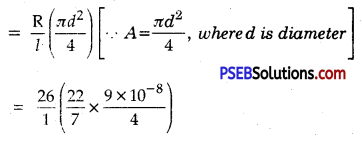= 1.84 × 10-6 Q m

Question 5.
From the given labeled circuit diagram, find :
(a) Resultant resistance of the circuit
(b) Current flowing in the circuit.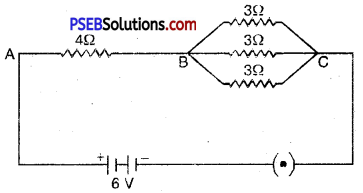(a) Suppose R’ is the equivalent resistance of the parallel arrangement of resistors 3Ω, 3Ω and 3Ω between points B and C, then ;
$$\frac{1}{\mathrm{R}^{\prime}}=\frac{1}{3}+\frac{1}{3}+\frac{1}{3}$$
= $$\frac{1+1+1}{3}$$
= $$\frac{3}{3}$$
∴ R’ = 1 Ω
Let R be the total resistance of series combination of 4 Ω between A and B and R’ = 1 Ω between B and C, then
R = 4Ω + R’
= 4 Ω + 1 Ω
∴ R = 5 Ω
Therefore, resultant resistance of the circuit is 5 Ω

(b) Now, potential difference of the battery (V) = 6V
and total resistance of the circuit (R) = 5 Ω
Current flowing in the circuit (I) = $$\frac{\mathrm{V}}{\mathrm{R}}$$
I = $$\frac{6 \mathrm{~V}}{5 \Omega}$$
I = 1.2 AQuestion 6.
(a) In an electric circuit, a battery of five cells each of 2V, resistors of 5 Ω, 10 Ω and 15 Ω and a key plug are connected in series arrangement. Draw its schematic diagram.
Schematic Diagram of the Circuit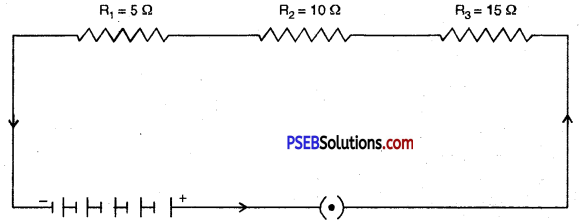(b) Calculate the value of current flowing in this circuit when plug is introduced in the key.
Three resistors of 5Ω, 10Ω and 15Ω when formed in series have resultant resistance R, then ;
R = R1 + R2 + R3
= 5 Ω + 10 Ω + 15 Ω
= 30 Ω
According to ohms law, I = $$\frac{\mathrm{V}}{\mathrm{R}}$$
I = $$\frac{10 \mathrm{~V}}{30 \Omega}$$
I = $$\frac{1}{3}$$A

Question 7.
An electric lamp, whose resistance is 20 Ω and a conductor of 4 Ω resistance are connected to a 6 V battery as shown in the figure. Calculate (a) total resistance of the circuit (6) the current through the circuit (c) the P.D. across the electric lamp and conductor.
Given : Resistance of lamp R1 = 20 Ω
and Resistance of conductor R2 = 4 Ω
Pot. difference of battery V = 6V
(a) ∵ both the resistances are connected in series,
∴ Total resistance of the circuit R = R1 + R2
= 20 Ω + 4 Ω
= 24 Ω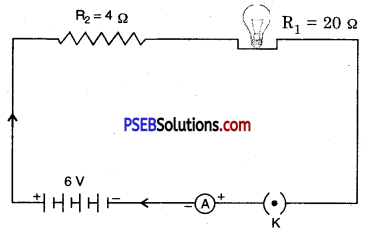(b) Total potential difference in the circuit V = 6 V
Total resistance in the circuit, R = 24 Ω
∴ Total current in the circuit, I = $$\frac{\mathrm{V}}{\mathrm{R}}$$
I = $$\frac{6 \mathrm{~V}}{24 \Omega}$$
I = 0.25 A

(c) Pot, difference across the lamp. V1 = 20 Ω × 0.25 A
V1 = 5V
and Pot, difference across the conductor, V2 = 4 Ω × 0.25 A
V2 = 1V

Question 8.
98 J of heat is produced each second in 2 resistor. Find the potential difference.
Here, H = 98 J
R = 2Ω
t = Is
Potential difference, V = ?
Using H = I2Rt
98J = I2 × 2Ω × 1s
or I2 = $$\frac{98}{2 \times 1}$$
I = $$\sqrt{49}$$
=7A
∴ 1= 7A

Thus, potential difference across the resistor,
V = I × R
V = 7A × 2 Ω
V = 14 volt.

Question 9.
The rating of an electric heater is 1100 W; 220V. Calculate the resistance when it operates at 220 V. Also calculate the energy consumed in kWh in the month of November, if the heater is used daily for 4 hours at the rated voltage.
Here P = 1100 W
V = 220 V
R =?
Number of days = 30
t = 4h × 30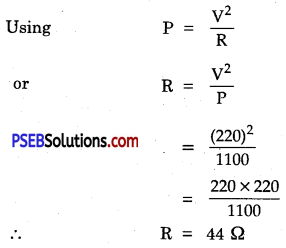Energy consumed by the heater in the month of November,
E = P × t
= 1100 W × (4 × 30 h)
= 132000 Wh
= 132 kWh

Question 10.
What is the (a) highest (b) lowest resistance that can be secured by combination of four Coils of resistance 4Ω, 8Ω, 10Ω and 20Ω?
(a) For highest resistance, resistors are connected in series :
R = R1 + R2 + R3 + R4
= 4Ω + 8Ω + 10Ω + 20 Ω = 42 Ω

(b) The lowest resistance will be obtained when these are connected in parallel.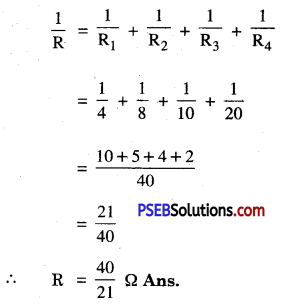Question 11.
An electric bulb of power 40 W is lighted daily for 8 hours for 15 days. How many units of electric energy will be consumed? Also find the amount of electric bill if the rate of electricity consumption is ₹ 8.00 per unit.
Given : Power, P = 40 W
Time each day = 8 hrs
No. of days = 15 days
∴ Total time for which bulb is lighted, t = 8 × 15 h
Now, electric energy consumed E = P × t
= 40 W × (8 × 15 h)
= 4800 Wh

∴ Electric energy consumed in units = $$\frac{4800}{1000}$$
= 4.8kWh
= 4.8 units

Amount of electric bill = No. of units × Rate
= 4.8 × 8.00
= ₹ 38.40Question 12.
A household uses the following electric appliances :
(а) The refrigerator of rating 400 W for 10 hours each day
(b) Two electric fans of rating 80 W each for 12 hours each day
(c) Six electric tubes of rating 18 W each for 6 hours each day! Calculate the electricity bill of the household for the month of April if the cost per unit of electric energy is ₹ 4.00
Electric energy consumed by all appliances working together each day = (400 × 10) + (2 × 80 × 12) + 6 × 18 × 6
= 4000 Wh + 1920 Wh + 648 Wh = 6568 Wh
= 6.568 kWh (units)
Total electric energy consumed in 30 days of April = 6.568 × 30 units
= 65.68 × 3
= 197.04

Electric Bill = 197.04 × 4
= ₹ 788.16

Question 13.
An electric motor takes 5 A current from 220 V line. Determine the power of the motor and energy consumed in 2 hours.
Given, V = 220 volt ; I = 5 A ; t = 2 hour = 7,200 s
Power consumed VI = 220 V × 5 A
= 1,100 watts
Vlt = 220V × 5 A × 2 h
= 2,200 Wh
= 2.2 kWh
or Energy consumed in S.I units = 1100 × 5 × 7200 s
= 3.96 × 107 J

Question 1.
Define energy.
Energy. It is the capacity to do work.

Question 2.
Define electric energy.
Electric Energy. Work done is the electric energy used to produce heat energy in an electric circuit.

Question 3.
Define electric current and state its unit.
Current. It is rate of flow of electric charge. Its unit is ampere.

Question 4.
Define a volt, whose unit is this?
Or
What is P.D.? Give SI unit.
P.D. (Volt) is work done in moving 1 coulomb of + ve charge from one point to other. It is the unit of potential difference. SI unit of P.D. is JC-1 or volt.

Question 5.
Show the switch signs in circuit in (i) open (ii) closed circuit.
Circuit Sign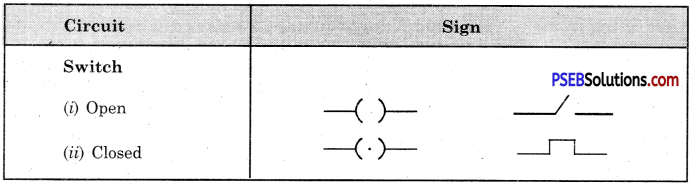Question 6.
Is electric potential a scalar or a vector quantity?
It is a scalar quantity.Question 7.
What is practical unit of power and electric energy?
Practical unit of power is watt and that of electric energy is kWh (kilo watt hour).

Question 8.
Which one is having more resistance, 100 W bulb or a 50 W bulb?
Resistance of 50 W bulb is twice that of 100 W bulb.

Question 9.
What constitutes the current?
Flow of free electrons constitute the current.

Question 10.
What is SI unit of resistivity?
It is ohm-m.

Question 11.
What is conductor of electricity? Give two examples.
Conductor of electricity. A substance that allows the electric current to pass through it is called conductor of electricity.

Examples :

• Copper
• Silver
• Human body.

Multiple Choice Questions:

Question 1.
V ∝ I law was given by:
(B) Watt
(C) Ohm
(D) Coulomb.
(C) Ohm

Question 2.
The unit of Potential is:
(A) Ampere
(B) Volt
(C) Ohm
(D) Watt.
(B) Volt

Question 3.
The unit of electric energy is:
(A) Ampere
(B) Volt
(C) Ohm
(D) Watt
(D) Watt

Question 4.
Resistance of a conductor depends on:
(A) its length
(B) its area of cross section
(C) nature of its material
(D) All of these.
(D) All of these.Question 5.
What maximum resistance can be obtained by combining three resistances each of ___________
(A) $$\frac{1}{3}$$ Ω
(B) 1Ω
(C) $$\frac{1}{9}$$ Ω
(D) 3Ω.
(B) 1Ω

Question 6.
By which unit electric current is represented?
(A) Coulomb
(B) Ampere
(C) Watt
(D) Kilowatt.
(B) Ampere

Question 7.
Electric current in circuits is measured by:
(A) Ammeter
(B) Voltmeter
(C) Galvanometer
(D) Electric meter.
(A) Ammeter

Question 8.
How is Ammeter always connected in circuits? :
(A) in series
(B) in parallel
(C) both in series and parallel
(D) None of these.
(A) in series

Question 9.
How is potential difference between two points expressed?
(A) V = $$\frac{\mathrm{W}}{\mathrm{Q}}$$
(B) Q = VW
(C) W = $$\frac{\mathrm{V}}{\mathrm{Q}}$$
(D) V = $$\frac{\mathrm{Q}}{\mathrm{W}}$$
(A) V = $$\frac{\mathrm{W}}{\mathrm{Q}}$$

Question 10.
How much work is done to carry 2 C of charge between two points?
(A) 2 J
(B) 6 J
(C) 24 J
(D) J.
(C) 24 J

Question 11.
According to ohm’s law:
(A) R = $$\frac{\mathrm{I}}{\mathrm{V}}$$
(B) R = $$\frac{\mathrm{V}}{\mathrm{I}}$$
(C) V = $$\frac{\mathrm{R}}{\mathrm{I}}$$
(D) I = $$\frac{\mathrm{V}}{\mathrm{R}}$$
(B) R = $$\frac{\mathrm{V}}{\mathrm{I}}$$

Fill in the blanks:

Question 1.
A stream of ________ moving through a conductor constitutes, electric current.
electrons.

Question 2.
The SI unit of electric current is ________
Ampere.

Question 3.
In an electric circuit ________ is always connected in parallel.